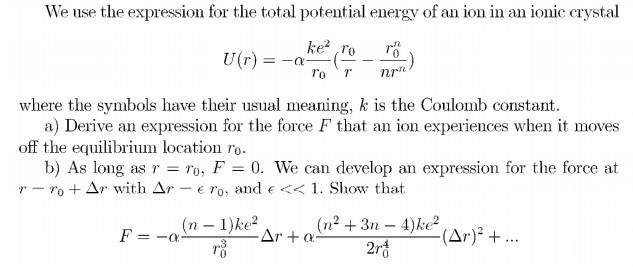# Solving Homework Equations: Taylor Series & Beyond

• cjw21

## Homework Statement## Homework Equations

All should be there, except taylor series, which is found here:

http://mathworld.wolfram.com/TaylorSeries.html

## The Attempt at a Solution

For part a, I got:

F(r)= $\alpha$(ke2)((-r0/r2)+(r0n/rn+1))

since force is the negative derivative of potential.

I'm pretty sure the answer to part b will involve a taylor expansion, but I'm not sure if the f(x) I use will be the equation I found in part a, or the derivative of the equation I found in part a. Additionally, I'm not sure how, in the answer we're supposed to get that's listed in the problem, they got (n-1) and the n term in the second part out of the exponent.

Thanks for any help!

sorry, double post